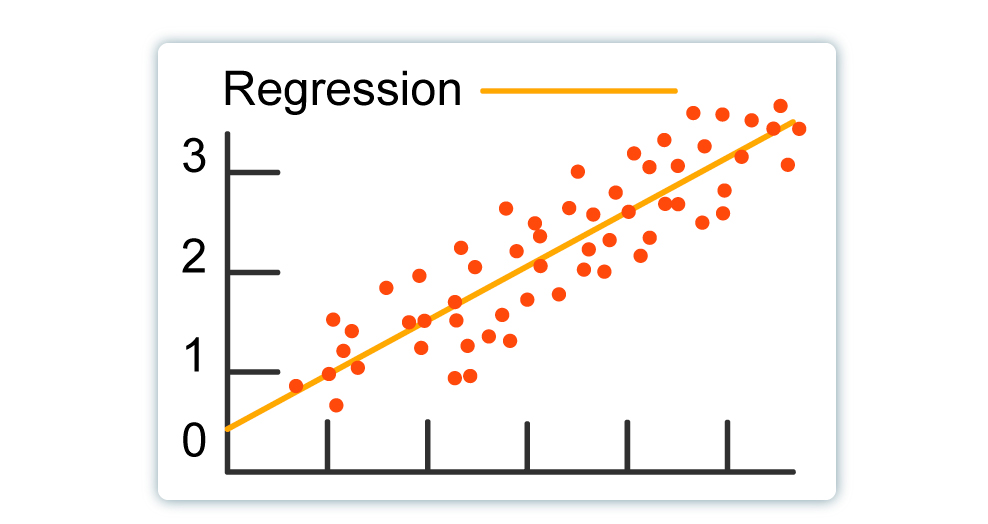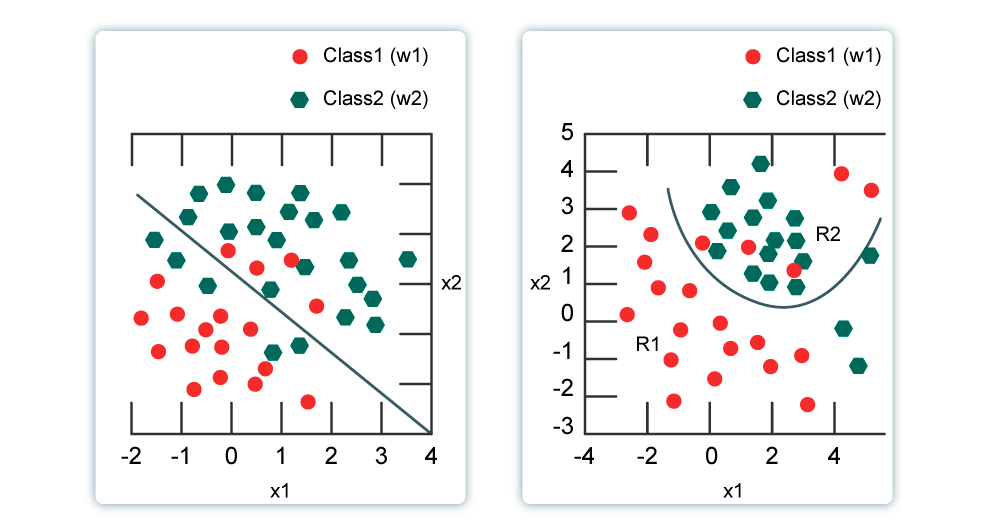# Machine LearningEvery day, machine learning takes an increasing place in our life due to the huge range of its applications. Starting from the analysis of traffic jams and ending with unmanned vehicles, more and more tasks are shifted to self-learning machines.

We sometimes have little idea how some applications based on machine learning methods work. For example, no one will be able to answer the question “Why did I see site A in the advertisement today, and not B?”. Most people have a misunderstanding about the principles of machine learning.

### Introduction

Machine learning is considered a branch of artificial intelligence, the main idea of ​​which is that the computer does not just use a pre-written algorithm, but learns how to solve the task.

Any working technology of machine learning can be conditionally attributed to one of the three levels of accessibility. The first level is when it is available only to different technology giants of the level of Google or IBM. The second level is when a student with a certain amount of knowledge can take advantage of it. The third level is when even an older person is able to cope with it.

Machine learning is now at the junction of the second and third levels, due to which the speed of changing the world with the help of this technology is growing every day.

Learning With A Teacher And Without A Teacher

Most of the tasks of machine learning can be divided into learning with the teacher (supervised learning) and learning without a teacher (unsupervised learning). By “teacher” is understood here the very idea of human intervention in the processing of data. When learning with a teacher, we have data on the basis of which we need to predict something, and some hypotheses. When learning without a teacher, we only have data whose properties we want to find. On the examples of the difference you will see a little more clearly.

### Learning With The Teacher

We have data on 10 000 apartments in a city, and the area of ​​each apartment, the number of rooms, the floor, the location, the availability of parking, the distance to the nearest metro station and so on is known. In addition, the cost of each apartment is known. Our task is to build a model that, based on these characteristics, will predict the cost of the apartment. This is a classic example of teaching with a teacher, where we have data (10,000 apartments and various parameters for each apartment, called signs) and responses (the cost of an apartment). This problem is called the regression problem. About what it is, we’ll talk later.Red dots are the available data (x is the sign value, y is the answer value), the yellow line is the constructed model.

Other examples: based on various medical indicators, predict the patient’s cancer. Or, based on the text of the email, it is possible to predict the likelihood that it is spam. Such tasks are classification tasks.The problem of classification. In the first picture, the objects are separated by a straight line. On the second more complex curve. Note that some objects are not classified correctly. This is a normal practice in classification problems.

### Learning Without A Teacher

The situation is more interesting with learning without a teacher, where “correct answers” are unknown. Let us know the data on the growth and weight of a certain number of people. It is necessary to group the data into 3 categories in order to produce a suitable size shirt for each category of people. This problem is called the clustering problem.Clustering on 3 clusters. Note that usually the separation into clusters is not so obvious and there is no single “correct” separation.

Another example is the situation where each object is described, say, by 100 signs. The problem with such data is that it is difficult to construct a graphic illustration of such data, so to say the least, we can reduce the number of signs to two or three. Then you can visualize the data on a plane or in space. Such a problem is called the diminishing problem.

### Classes Of  Tasks Of Machine Training

In the previous section, we gave several examples of machine learning tasks. In this we will try to generalize the categories of such problems, accompanied by a list of additional examples.

• The problem of regression: based on various characteristics, predict the real answer. In other words, the answer can be 1, 5, 23.575 or any other real number, which, for example, can represent the cost of an apartment. Examples: predicting the value of the stock in six months, predicting the store’s profit next month, predicting the quality of wine on blind testing.
• The task of classification: on the basis of various characteristics to predict the categorical response. In other words, there are a finite number of answers in this task, as in the case of determining the patient’s cancer or determining whether the letter is spam. Examples: recognition of text by handwriting, determining whether a person or a cat is in the photo.
• The task of clustering: splitting data into similar categories. Examples: a breakdown of the cellular operator’s customers by paying capacity, breaking up space objects into similar ones (galaxies, planets, stars and so on).
• The problem of diminishing dimensionality: to learn how to describe our data not with N signs, but with a smaller number (usually 2-3 for subsequent visualization). As an example, in addition to the need for visualization, you can result in data compression.
• The problem of detecting anomalies: on the basis of signs, one can learn to distinguish between anomalies and “non-anomalies”. It seems that this task does not differ from the classification problem. But the peculiarity of revealing anomalies is that we have either very few examples of anomalies for training the model, or not at all, so we can not solve such a problem as a classification problem. Example: identification of fraudulent transactions on a bank card.

### Neural Networks

In machine learning, there are a large number of algorithms, and some are quite universal. Examples include the support vector method, the decision-making on deciding trees or the same neural networks. Unfortunately, most people vaguely imagine the essence of neural networks, attributing to them properties that they do not possess.

A neural network (or an artificial neural network) is a network of neurons, where each neuron is a mathematical model of a real neuron. Neural networks began to enjoy great popularity in the 80’s and early 90’s, but in the late 90’s their popularity fell dramatically. However, recently this is one of the advanced technologies used in machine learning, used in a huge number of applications. The reason for the return of popularity is simple: the computing capabilities of computers have increased.

With the help of neural networks it is possible to solve at least regression and classification problems and build extremely complex models. Without going into mathematical details, one can say that in the middle of the last century Andrei Kolmogorov proved that using a neural network one can approximate any surface with any accuracy.

In fact, a neuron in an artificial neural network is a mathematical function (for example, a sigmoid function), to which some value comes to the input and a value obtained using the same mathematical function is obtained at the output.

### Limits Of Neural Networks

However, in neural networks there is nothing magical, and in most cases, concerns about the scenario of the “Terminator” have no basis. Let’s say scientists have trained a neural network to recognize handwritten digits (such an application can use, say, mail). How can such an application work and why is there nothing to worry about?

Let’s say we work with images of 20×20 pixels, where each pixel is represented by a shade of gray (only 256 possible values). As a response, we have one of the numbers: from 0 to 9. The structure of the neural network will be as follows: in the first layer there will be 400 neurons, where the value of each neuron will be equal to the intensity of the corresponding pixel. In the last layer there will be 10 neurons, where in each neuron there will be a probability that the corresponding figure is drawn on the original image. Between them there will be a certain number of layers (such layers are called hidden) with the same number of neurons, where each neuron is connected to a neuron from the previous layer and with no more.The edges of the neural network (in the picture they are shown as arrows) will correspond to some numbers. And the value in the neuron will be considered as the following sum: the value of the neuron from the previous layer * the value of the edge connecting the neurons. Then a certain function (for example, the sigmoid function, about which we spoke earlier) is taken from this sum.

Ultimately, the task of training a neural network is to select such values in the edges to give the first layer of the neural network the intensity of the pixels, on the last layer we get the probabilities that a certain number is drawn on the image.

In simpler words, in this case the neural network is a calculation of a mathematical function, where arguments are other mathematical functions that depend on other mathematical functions and so on. Of course, with such a calculation of mathematical functions, where certain arguments are fitted, there can not be any existential risk of speech.

### Interesting Facts And Lifehakes

Here are a few interesting and not very obvious examples of using machine learning in real life.

For example, the second campaign of Barack Obama was in fact won by the best team in the field of data analysis at that time. Of course, we are not talking about the fact that they advised him to lie about something, the work was built in a much more intelligent way: they chose in which state, in front of which audience, on what day and on what topic he should speak. And each time they measured how it affects the polls of the kind “Who would you vote for, if the elections were on the next Sunday?”. In other words, such decisions were made not by politicians, but by specialists in data analysis. It becomes especially interesting in the light of the fact that, according to experts, this gave him an advantage of 8-10%.

In addition, modern Internet is quite difficult to imagine without retargeting, or personalized advertising. Remember: you choose a product on the Internet, and after buying for another two weeks you show it in various kinds of advertising.